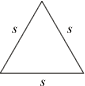# Equilateral Triangle Area Calculator

This calculator requires the use of Javascript enabled and capable browsers. This calculator is designed to give the area of an equilateral triangle, assuming a side length is known. Enter the side. Then click on calculate. In a triangle, all interior angles total to 180 degrees. No two angles can total to 180 degrees or more; in an equilateral triangle, all angles are equal, or 180 degrees/3. The formula for the area is the square root (3)/4 * (s * s). It is for equilateral triangles. In case you need them, here are the Trig Triangle Formula Tables, as well as the Right Triangle Angle And Side Calculator.

 Unit Number Required Data Entry Known Equilateral Side Length Units Calculated Results Area Square Units Derived Angles DegreesUpdated 8.12.11

 Leave us a question or comment on FacebookSearch or Browse Our Site